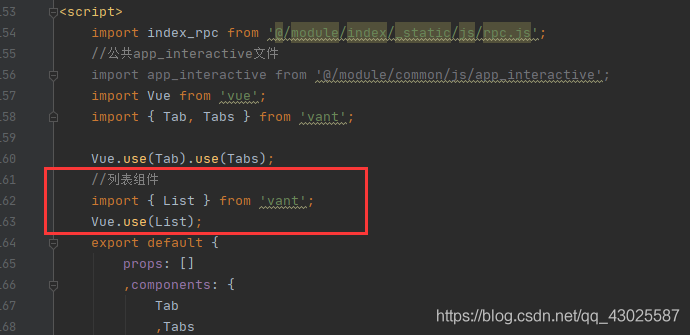vue.js### 问题描述

```this.currentPage = 1;
//每次走完函数，将当前页恢复至1，防止后面累加，导致点击别的筛选条件时无效，返回finished-text
this.finished = false;
//同样，也要将finished恢复，否则，执行别的筛选条件时，会显示finished-text,并且导致明明还有数据,但是不会加载出来.```

```<van-list
:finished="finished"
finished-text="我是有底线的~"
<van-row
v-for="(item,key) of Array"
:key="key"
</van-row>//循环，显示列表
</van-list>
```
```selType(type) {//从前端的点击事件获得type
this.selectedType = type;
console.log(this.selectedType);
this.currentPage = 1;
//每次走完函数，将当前页恢复至1，防止后面累加，导致点击别的筛选条件时无效，返回finished-text
this.finished = false;
//同样，也要将finished恢复，否则，执行别的筛选条件时，会显示finished-text,并且导致明明还有数据,但是不会加载出来.
},
this.search();
}

search() {//调用查询的接口
let params = {
type: this.selectedType,
index: this.currentPage,//页数
size: this.pageSize,//每页个数
}
.....（接口）(params).then(res => {
console.log(res);
this.dataTotal = res.total;
//进行判断
if(this.dataTotal <= this.pageSize){
this.Array = res.data.list;
console.log(this.Array);
}else{
this.currentPage++;
let arr = res.data.data.list;
this.Array = this.Array.concat(arr);
};
// 加载状态结束
// 数据全部加载完成
if (this.Array.length >= this.dataTotal) {
this.finished = true;//结束，显示我也是有底线的
};
});
}
```

## vant van-list的使用及一些坑的解决

### 第二步

```import Vue from ‘vue‘;
import { List } from ‘vant‘;

Vue.use(List);
```### 第三步### 第四步

js中重要代码

```data(){
return{
content_list: [],
finished: false,//是否加载
count_page: 1,//加载页数
count_num: 4//每页限制条数
}
},
methods:{
var _this = this;
_this.count_page += 1;
// 请求数据
_this.get_course_list();
}
，get_course_list:function(){
var _this = this;
var params ={};
params.page = _this.count_page;
params.num = _this.count_num;
index_rpc.get_collection_course(params,function (data) {
if(data.error_code == 0) {
var item = data.data || [];
_this.count = data.count;
if (params.count_page == 1){
_this.content_list = [];
}
item.forEach(function(val) {
_this.content_list.push(val);
})
//这里是用于判断什么时候所有数据加载完毕，然后进行是否进行加载关闭
if(_this.count_num * _this.count_page >= _this.count) {
_this.finished = true;
}else {
_this.finished = false;
}
}else{
_this.count = 0;
_this.content_list = [];
_this.finished = true;
}
})
}
}
```